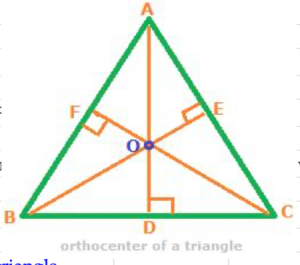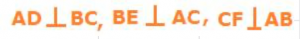## Orthocenter definition.

Orthocenter is defined as the point of intersection of the altitudes of a triangle.Consider the above triangle ABC, in which BC, AC and AB are the three sides. With respect to the three sides BC, AC and AB taken as bases, the three straight lines AD, BE and CF are the heights of the triangle. Heights of a triangle are also called altitudes of the triangle. Altitudes of a triangle are perpendicular to the respective bases at the points where they meet the latter.

In the above triangle ABC,The three altitudes, AD, BE and CF intersect each other at the point O, called the orthocenter of the triangle.

## Orthocenter questions in coordinate geometry

There can be three types of Orthocenter questions normally asked in coordinate geometry. They are:

1. Find orthocenter when centrod, O and circumcenter, S are given.
2. Find orthocenter of a right angle triangle, the coordinates of whose three vertices are given.
3. Find orthocenter of an equilateral triangle.

### Solution to question 1

In a triangle, let S, G and O denote Circumcenter, Centroid and Orthocenter respectively. First of all, S, G and O are collinear points, i.e. all three of S, G and O lie on a same straight line. Next, the Centroid G divides the line joining the three points S, G and O in the ratio 1: 2, i.e SG: GO = 1: 2. If the coordinates of any two of the three points S, G and O are given, then the coordinates of the third point can be found using the section formula of division.

### Solution to question 2

#### Example:

The coordinates of the three vertices of a right angled triangle are (2, 6) (2, 4) and (3, 4). Find the orthocenter of the triangle.

#### Solution:

In a right angled triangle, the vertex where the right angleĀ is formed is the orthocenter of the triangle. Which of the three points will lie at the vertex containing the right angle? Among the three points given, the point which shares a same x coordinate and y coordinate with the other two points will lie at the vertex of the right angled triangle. In the three points A (2, 6) B (2, 4) and C (3, 4), The point B (2, 4) shares a common x coordinate with point A and a common y coordinate with the point C. Therefore, the point B (2, 4) will lie at the vertex containing the right angle. So, B (2, 4) is the orthocenter of the right angled triangle.